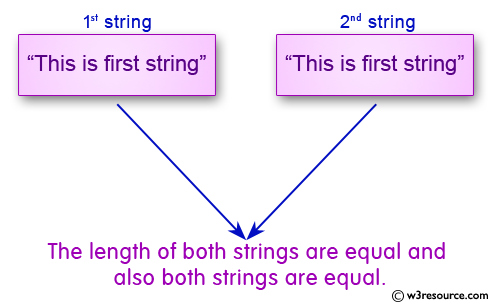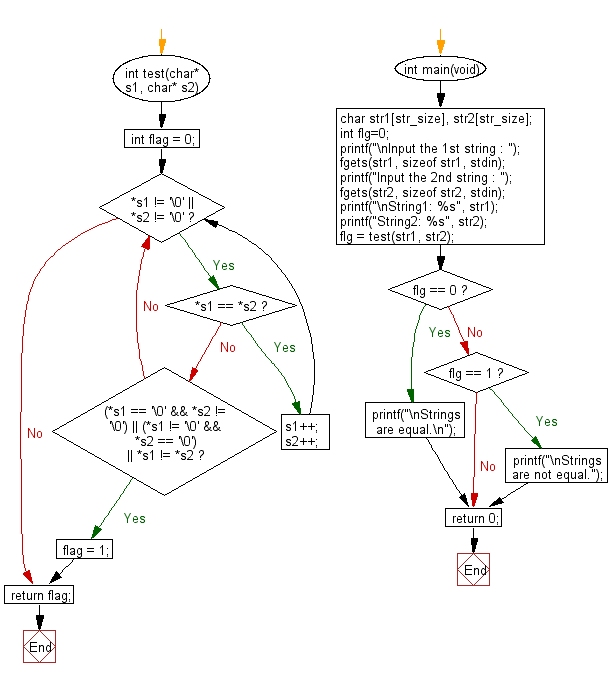﻿ C exercises: Compare two string whether they are equal or not - w3resource# C Programming: Compare two string whether they are equal or not

## C String: Exercise-6 with Solution

Write a program in C to compare two string without using string library functions.Sample Solution:

C Code:

``````#include <stdio.h>
#include <string.h>
#include <stdlib.h>

#define str_size 100 //Declare the maximum size of the string

void main()
{
char str1[str_size], str2[str_size];
int flg=0;

printf("\n\nCompare two string whether they are equal or not :\n");
printf("------------------------------------------------------\n");
printf("Input the 1st string : ");
fgets(str1, sizeof str1, stdin);

printf("Input the 2nd string : ");
fgets(str2, sizeof str2, stdin);

int i=0;

/* Runs till both strings are equal */
while(str1[i] == str2[i])
{
if(str1[i] == '\0' || str2[i] == '\0')
break;

i++;
}
if(str1[i-1] == '\0' && str2[i-1]=='\0')
flg=0;
else if(str1[i] > str2[i])
flg=1;
else if(str1[i] < str2[i])
flg=-1;

if(flg == 0)
{
printf("\nThe length of both strings are equal and \nalso both strings are equal.\n\n");
}
else if(flg == -1)
{
printf("\nThe length of the first string is smaller than second.\n\n");
}
else
{
printf("\nThe length of the first string is greater than second.\n\n");
}
}
```
```

Sample Output:

```Compare two string whether they are equal or not :
------------------------------------------------------
Input the 1st string : This is first string
Input the 2nd string : This is first string

The length of both strings are equal and
also both strings are equal.
```

Flowchart:C Programming Code Editor:

Improve this sample solution and post your code through Disqus.

What is the difficulty level of this exercise?

﻿